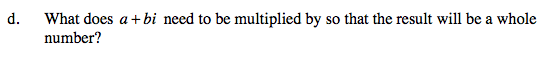Home > PC > Chapter 11 > Lesson 11.1.3 > Problem11-48

11-48.
1. Multiply each of the following complex numbers. Recall that i =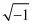and i2 = −1. Homework Help ✎

1. (3 + 2i) (3 − 2i)

2.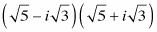3. What does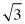+ 7i need to be multiplied by so that the result will be a whole number?

4. What does a + bi need to be multiplied by so that the result will be a whole number?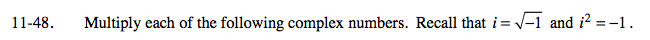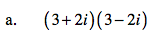13
Did you remember to use the Distributive Property?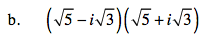$(\sqrt{5})(\sqrt{5})+(\sqrt{5})(\textit{i}\sqrt{3})-(\textit{i}\sqrt{3})(\sqrt{5})-(\textit{i}\sqrt{3})(\textit{i}\sqrt{3})$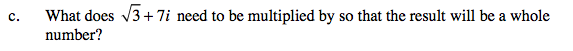The answers for parts (a) and (b) are both whole numbers. How are the factors being multiplied the same or different?